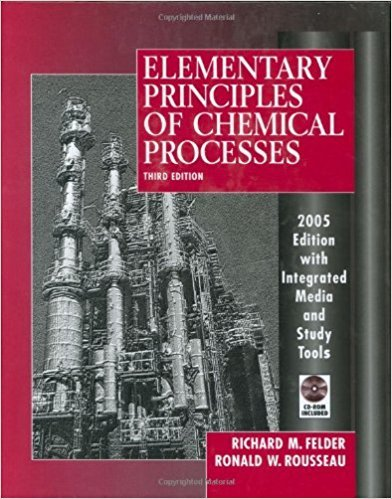×
Get Full Access to Elementary Principles Of Chemical Processes - 3 Edition - Chapter 7 - Problem 7.9
Get Full Access to Elementary Principles Of Chemical Processes - 3 Edition - Chapter 7 - Problem 7.9

×

# Write and simplify the closed-system energy balanceISBN: 9780471687573 143

## Solution for problem 7.9 Chapter 7

Elementary Principles of Chemical Processes | 3rd Edition

• Textbook Solutions
• 2901 Step-by-step solutions solved by professors and subject experts
• Get 24/7 help from StudySoup virtual teaching assistantsElementary Principles of Chemical Processes | 3rd Edition

4 5 1 333 Reviews
10
3
Problem 7.9

Write and simplify the closed-system energy balance (Equation 7.3-4) for each of the following processes, and state whether nonzero heat and work terms are positive or negative. Begin by defining the system. The solution of part (a) is given as an illustration. (a) The contents of a closed flask are heated from 25C to 80C. Solution. The system is the flask contents. Q- W = t::.u + t::.Ek + t::.Ep W = 0 (no moving parts or generated currents) I'1Ek = 0 (system is stationary) I'1Ep = 0 (no height change) I Q = t::.u I Q > 0 (heat is transferred to the system) (b) A tray filled with water at 20C is put into a freezer. The water turns into ice at - 5~C. (Note: When a substance expands it does work on its surroundings and when it contracts the surroundings do work on it.) (c) A chemical reaction takes place in a closed adiabatic (perfectly insulated) rigid container. (d) Repeat part (c), only suppose that the reactor is isothermal rather than adiabatic and that when the reaction was carried out adiabatically the temperature in the reactor increased

Step-by-Step Solution:
Step 1 of 3

Step 2 of 3

Step 3 of 3

##### ISBN: 9780471687573

The full step-by-step solution to problem: 7.9 from chapter: 7 was answered by , our top Chemistry solution expert on 11/15/17, 02:42PM. This textbook survival guide was created for the textbook: Elementary Principles of Chemical Processes, edition: 3. The answer to “Write and simplify the closed-system energy balance (Equation 7.3-4) for each of the following processes, and state whether nonzero heat and work terms are positive or negative. Begin by defining the system. The solution of part (a) is given as an illustration. (a) The contents of a closed flask are heated from 25C to 80C. Solution. The system is the flask contents. Q- W = t::.u + t::.Ek + t::.Ep W = 0 (no moving parts or generated currents) I'1Ek = 0 (system is stationary) I'1Ep = 0 (no height change) I Q = t::.u I Q > 0 (heat is transferred to the system) (b) A tray filled with water at 20C is put into a freezer. The water turns into ice at - 5~C. (Note: When a substance expands it does work on its surroundings and when it contracts the surroundings do work on it.) (c) A chemical reaction takes place in a closed adiabatic (perfectly insulated) rigid container. (d) Repeat part (c), only suppose that the reactor is isothermal rather than adiabatic and that when the reaction was carried out adiabatically the temperature in the reactor increased” is broken down into a number of easy to follow steps, and 190 words. Elementary Principles of Chemical Processes was written by and is associated to the ISBN: 9780471687573. Since the solution to 7.9 from 7 chapter was answered, more than 871 students have viewed the full step-by-step answer. This full solution covers the following key subjects: system, closed, work, reactor, surroundings. This expansive textbook survival guide covers 13 chapters, and 710 solutions.

Unlock Textbook Solution